• 2021-10-13 16:20:45

通常，在程序设计中，我们会发现一些程序段在程序的不同地方反复出现，此时可以将这些程序段作为相对独立的整体，用一个标识符给它起一个名字，凡是程序中出现该程序段的地方，只要简单地写上标识符即可。这样的程序段，我们称之为子程序（函数）。

C++常用函数有哪些？

C++常用函数有如下这些：
函数名：memchr

函数原型：voidmemchr(constvoidbuf，intc，sizetcount)；

参数：buf缓冲区的指针；c查找的字符；count检查的字符个数。

所需头文件：

功能：查找buf的前count个字节中c的第一次出现，当找到c或已检查完count个字节时停止。

返回值：如果成功，返回buf中c首次出现的位置的指针；否则返回NULL

函数名：memcpy

函数原型：voidmemcpy(voiddest，constvoid*src，sizetcount)；

参数：dest目的缓冲区；src源缓冲区；count拷贝的字符个数。

所需头文件

功能：从src拷贝count个字节到dest。如果源缓冲区和目的缓冲区重叠，这个函数不能保证正确拷贝；对于这种情况可使用memmove处理。

返回值：返回dest的值。

函数名：memmove

函数原型：voidmemmove(voiddest，constvoid*src，sizetcount)；

参数：dest目的对象；src源对象；count拷贝的字符字节个数。

所需头文件；

功能：从src拷贝count个字节到dest。如果源区域与目的区域有重叠，memmove也能确保正确拷贝。

返回值：返回dest的值。c语言
更多相关内容
• XML在Json出现前应用很广泛，灵活性好，应用语言也没有限制，发展了这么长时间后xml标准已经很臃肿。这篇文章主要介绍了C++常用函数之XML JSON格式转换问题,需要的朋友可以参考下
• 可以查询常用函数，中文版本，带解释，免费下载，希望大家有所收获。
• 注：写这篇文章是为了平时学习积累噢！文中的内容都表明出处啦，我只写了大概，不如原博主写的好，大家见谅哈~ 目录vectoraccumulate ...2、Vector作为函数的参数或者返回值时，需要注意它的写法： double Distan

注：写这篇文章是为了平时学习积累噢！文中的内容都表明出处啦，我只写了大概，不如原博主写的好，大家见谅哈~

## vector

作用：它能够像容器一样存放各种类型的对象，简单地说，vector是一个能够存放任意类型的动态数组，能够增加和压缩数据。

特别注意：

使用vector需要注意以下几点：

1、如果你要表示的向量长度较长（需要为向量内部保存很多数），容易导致内存泄漏，而且效率会很低；

2、Vector作为函数的参数或者返回值时，需要注意它的写法：

double Distance(vector&a, vector&b) 其中的“&”绝对不能少！！！

实例：vectortest;

//建立一个vector，int为数组元素的数据类型，test为动态数组名

简单的使用方法如下：

vector<int>test;//建立一个vector

test.push_back(1);

test.push_back(2);//把1和2压入vector，这样test就是1,test就是2

vector<vector<Point2f> > points; //定义一个二维数组

points.size();  //指第一行的列数


基本操作

(1)头文件#include.

(2)创建vector对象，vector vec;

(3)尾部插入数字：vec.push_back(a);

(4)使用下标访问元素，cout<<vec<<endl;记住下标是从0开始的。

(5)使用迭代器访问元素.

vector<int>::iterator it;

for(it=vec.begin();it!=vec.end();it++)

cout<<*it<<endl;



(6)插入元素： vec.insert(vec.begin()+i,a);在第i+1个元素前面插入a;

(7)删除元素： vec.erase(vec.begin()+2);删除第3个元素

vec.erase(vec.begin()+i,vec.end()+j);删除区间[i,j-1];区间从0开始

(8)向量大小:vec.size();

(9)清空:vec.clear();
算法

• 使用reverse将元素翻转：需要头文件 #include<algorithm>

reverse(vec.begin(),vec.end());将元素翻转，即逆序排列！

(在vector中，如果一个函数中需要两个迭代器，一般后一个都不包含)

• 使用sort排序：需要头文件#include，

sort(vec.begin(),vec.end());(默认是按升序排列,即从小到大).

可以通过重写排序比较函数按照降序比较，如下：

定义排序比较函数：

bool Comp(const int &a,const int &b)
{
return a>b;
}


调用时:sort(vec.begin(),vec.end(),Comp)，这样就降序排序。

• 输出Vector的中的元素
vector<float> vecClass;

int nSize = vecClass.size();


//打印vecClass,方法一：

for(int i=0;i<nSize;i++)
{
cout<<vecClass[i]<<"     ";
}
cout<<endl;


需要注意的是：以方法一进行输出时，数组的下标必须保证是整数。
//打印vecClass,方法二：

for(int i=0;i<nSize;i++)
{
cout<<vecClass.at(i)<<"     ";
}
cout<<endl;


//打印vecClass,方法三：输出某一指定的数值时不方便

for(vector<float>::iterator it = vecClass.begin();it!=vecClass.end();it++)
{
cout<<*it<<"   ";
}
cout<<endl;


二维数组的使用：

#include "stdafx.h"
#include <cv.h>
#include <vector>
#include <iostream>
using namespace std;
int main()
{
using namespace std;
int out = { 1, 2,
3, 4,
5, 6 };
vector <int*> v1;

v1.push_back(out);
v1.push_back(out);
v1.push_back(out);

cout << v1 << endl;//1
cout << v1 << endl;//2
cout << v1 << endl;//3
cout << v1 << endl;//4
cout << v1 << endl;//5
cout << v1 << endl;//6

return 0;
}


转自博主 那年聪聪

使用const引用是一个好习惯(const 修饰容器，则初始化后不能增删元素也不能修改元素，也不能改变容器大小 ,也就说不能做任何改动。）
在C++中容器都是值拷贝的，因此不使用引用的话，会大量拷贝垃圾数据.其实，可以这么说C/C++是值拷贝编程语言。不使用额外的技术，比如指针、引用，都会发生垃圾数据的拷贝。当然，到底是不是垃圾数据由你自己决定，值拷贝是无法彻底摒弃的。

c++中vector和vector<int*>的用法比较（转自 军说网事）

## accumulate

accumulate定义在#include<numeric>中，作用有两个，一个是累加求和，另一个是自定义类型数据的处理

• 算法简介：accumulate(iterator beg, iterator end, value);
计算容器元素累计总和；[beg,end)为起始迭代器和结束迭代器，vaule为起始值 。

主要用于数值的累加求和、字符串的连接。

例子
使用accumulate(iterator beg, iterator end, value)函数计算vector容器中所有元素（0-100）的和，其初始值为1000

#include<iostream>
#include<vector>
#include<string>
#include<numeric>
using namespace std;

// 常用算术生成算法: accumulate(iterator beg, iterator end, value); 计算[beg,end)区间中所有元素的和
// all = value + sum(beg,end)

void test01(){

vector<int> vec;
for(int i=0; i<=100; i++){
vec.push_back(i);
}

vector<string> vecs;
vecs.push_back("Hello");
vecs.push_back("World");
vecs.push_back("!");

// 对string类型进行累积
string sunStr = accumulate(vecs.begin(), vecs.end(), string(""));

// 计算vec容器中所有元素的总和
int sumNum = accumulate(vec.begin(), vec.end(), 1000);

cout << "vec容器中元素的总和为：" << sumNum << endl;
cout << "vecs为:" << sunStr << endl;

}

int main(){

test01();

system("pause");
return 0;

}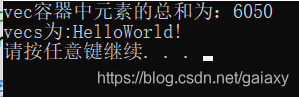转自博主 凉冰难消一腔热血
构建二维动态数组

int **p;
p = new int*;    //注意，int*表示一个有10个元素的指针数组
for (int i = 0; i < 10; ++i)
{
p[i] = new int;
}


定义二维vector

vector<vector<int>> v;


访问二维vector的元素的三种方式
如果指定外层和内层向量的大小，就可用operator[]进行读和写；如果只指定外层向量大小，就能用push_back()函数进行写，不能用operator[]进行读和写。

1. 指定外层vector大小

可用push_back函数进行初始化：

v.resize(3);
v.push_back(9);

1. 遍历指定内层vector大小
提前设定好每行vector的大小，就可用operator[]访问，如下：
for(int i=0;i<3;i++)
v[i].resize(3);

1. 一次指定内外层vector大小
v.resize(n, vector<int>(m));


定义一个二维整形数组并初始化：

vector<vector<int> > array(m); //这个m一定不能少//vector<int> array[m];
//初始化一个m*n的二维数组
for(int i=0;i<m;i++)
{
array[i].resize(n);
}
//遍历赋值
for(i = 0; i < array.size(); i++)
{
for (j = 0; j < array.size();j++)
{
array[i][j] = (i+1)*(j+1);
}
}


## sort

用法：

1、sort函数可以三个参数也可以两个参数，必须的头文件#include < algorithm>;

2、它使用的排序方法是类似于快排的方法，时间复杂度为n*log2(n)

3、sort函数有三个参数：（第三个参数可不写）
（1）第一个是要排序的数组的起始地址。
（2）第二个是结束的地址（最后一位要排序的地址）
（3）第三个参数是排序的方法，可以是降序也可是升序；还可以不写第三个参数，此时默认的是升序。

两个参数：

#include<iostream>
#include<algorithm>
using namespace std;

int main(){
int a={1,3,5,2,14,6,7,62,9};
for(int i=0;i<10;i++){
sort(a,a+10);
for(i=0;i<10;i++){
cout<<a[i]<<" ";
}
}
system("pause");
return 0;

}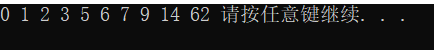三个参数：

#include<iostream>
#include<vector>
#include<algorithm>
using namespace std;

bool myfunction(int i,int j){
return (i<j);
}//升序排列
bool myfunction2(int i,int j){
return (i>j);
}//降序排列
struct myclass{
bool operator()(int i,int j){
return (i<j);
}
}myobject;

int main(){

int myints={32,14,64,3,23,6,44,75};
vector<int> myvector(myints,myints+8);

sort(myvector.begin(),myvector.begin()+4);
sort(myvector.begin()+4,myvector.end(),myfunction);
sort(myvector.begin(),myvector.end(),myobject);

cout<<"myvector contains:";
for(vector<int>::iterator it=myvector.begin();it!=myvector.end();it++){
cout<<' '<<*it;
}
cout<<'\n';

system("pause");
return 0;

}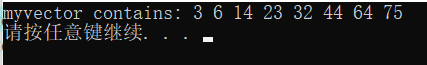string使用反向迭代器来完成逆序排列（rbegin,rend)

#include<iostream>
using namespace std;

int main(){
string str("abcdef");
string s(str.rbegin(),str.rend());
cout<<s<<endl;

return 0;

}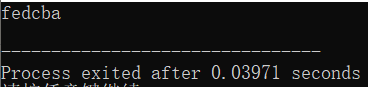转自博主 布林叮咚

想要拷贝元素：for(auto x:range)

想要修改元素 : for(auto &&x:range)

想要只读元素：for(const auto &x:range)

## find

find() 函数本质上是一个模板函数，用于在指定范围内查找和目标元素值相等的第一个元素。

如下为 find() 函数的语法格式：
InputIterator find (InputIterator first, InputIterator last, const T& val);

其中，first 和 last 为输入迭代器，[first, last) 用于指定该函数的查找范围；val 为要查找的目标元素。

展开全文• 数据类型转换，sort()排序函数，求最大公约数，字符串函数，vector、map容器

### 数据类型的转换

#include<iostream>
#include<sstream>
using namespace std;

//整形转化为字符串型，其余类推
string itos(int n) {
string s;
sstringstream ss;
ss<<n;
ss>>s;
return s;
}

int main()
{
int n = 1024;
string s = itos(n);
for(int i=0; i<s.length(); i++) {
cout<<s[i]<<",";
}
cout<<endl;

return 0;
}


### 排序函数

#include<algorithm>
#include<iostream>
using namespace std;

//设置排序方式函数
bool compare(int a, int b) {
return a>b;
}

int main()
{
int a[] = {1,3,2,5,34,3,5,6};
sort(a,a+20,compare);
}



### 比较字符串内容

#include<iostream>
#include<string.h>  //or #include<cstring>
using namespace std;

int main()
{
char *a = "11112";
char *b = "11117";
cout<<strcmp(a,b)<<endl;
return 0;

//a==b -> 返回 0；
//a>b -> 返回大于0的数；
//a<b -> 返回小于0的数；
}



### 辗转相除法求最大公约数

int fun(int m,int n){
int rem;			//余数，当余数为0的时候，最后的m即为最大公约数
//先用较小的数对较大的数取余，再用余数对较小的数求余，直到余数为零
while(n > 0){
rem = m % n;
m = n;
n = rem;
}
return m;			//将结果返回
}


### vector 容器

#include<iostream>
#include<string.h>
#include<vector>
#include<algorithm>

using namespace std;

int main()
{
vector<int> n;      //新建容器，类型为int

n.push_back(1024);      //向容器内添加元素

n.pop_back();       //移除最后一个数据

cout<<n.size()<<endl;      //输出n中数据的数量

n.clear();      //清空n中所有的元素

sort(n.begin(),n.end());        //从小到大排序
reverse(n.begin(),n.end());     //从大到小排序       排序所使用的sort函数可重写

for(int i=0; i<n.size(); i++) {
cout<<n<<endl;
}                                      //访问n中的元素

return 0;
}


### map容器

#include<map>
#include<iostream>
using namespace std;

int main()
{
map<char, int>mapstudent;       //构建map容器

mapstudent.insert(pair<char,int>('wang',803));      //插入数据
mapstudent['li']=801;          //简单的map数据插入

mapstudent.find('aaa');

cout<<mapstudent['wang']<<endl;     //根据键查找相应的值,若没有该键，则在map中添加此键

int a = mapstudent.size();      //查看map中数据的数量
cout<<a<<endl;

char key = 'wang';          //判断map中是否含有该键，若有则输出对应的值，没有则不做操作
if(mapstudent.count(key)>0) {
cout<< mapstudent[key]<<endl;;
}

int b = mapstudent.erase('li');     //删除元素，删除成功返回1，否则返回0
cout<<b<<endl;

return 0;

}

展开全文算法 数据结构 经验分享 程序人生
• 主要介绍了C/C++常用函数易错点分析,包含了memset、sizeof、getchar三个常用函数的分析,需要的朋友可以参考下
•文档
• ## C/C++常用函数合集

万次阅读 多人点赞 2018-09-03 22:53:46
参数解释 is 进行读入操作的输入流，常用cin str 已声明的用于存储读入内容的对象，常为string类型 delim 终结符 作用：将输入流is中读到的字符存入str中，直到遇到终结符delim才结束。如果没有定义delim，则默认为...

目录

C标准库

<string.h>头文件

2.memcpy()

3.strcpy()

4.strncpy()

5.strcat()

6.getline()

7.strlen()

<ctype.h>头文件

1.tolower()/toupper()

2.isalpha（），isdigit（），isprint（）

<math.h>头文件

1.pow()

2.floor()

3.ceil()

4.atan()

STL

<algorithm>头文件

1.min(),max()函数

2.lower_bound()

3.upper_bound（）

4.next_permutation()/prev_permutation()

6.Sort()

8.reverse()

# <string.h>头文件

## 1.memset()

函数原型：memset(void *s , int c , size_t n)

作用：将已开辟内存空间s的首n个字节的值设置为c。一般用于在对字符串进行初始化为‘\0’或‘ ’

注意：1.s为首地址，c为要赋予的字符，n为长度

2.一般不用于初始化数字数组

代码示例：

#include<stdio.h>
#include<string.h>

int main(){
char str={'1','2','3','4','5'};

printf("原始字符数组:");
for(int i=0;i<5;i++)
printf("%c ",str[i]);
printf("\n");

//	内存初始化
memset(str,'\0',5);

printf("初始化后的字符数组:");
for(int i=0;i<5;i++)
printf("%c ",str[i]);

return 0;
}

## 2.memcpy()

函数原型：void *memcpy(void *dest, const void *src, size_t n);

作用：将以src开头，长度为n的内存空间里的内容拷贝到以dest开头的内存空间里去。

例：

char a,b;
memcpy(b,a,sizeof(b)); //将a的sizeof(b)个元素赋给b

注意：如果用sizeof(a),会造成b的内存地址溢出。

## 3.strcpy()

函数原型：char *strcpy(char* dest, const char *src);

作用：把从src地址开始且含有NULL结束符的字符串复制到以dest开始的地址空间

例：

char a,b;
strcpy(a,b);

注意：遇到‘\0’时就结束拷贝

## 4.strncpy()

函数原型：strncpy(char* dest,const char* src,int n)

作用：把src所指的字符串中以src地址开始的前n个字节复制到dest所指的空间中，并返回dest

注意：1.结果dest不包括结束符NULL（'\0'）

2.如果src的长度小于n个字节，则以NULL填充dest直到复制完n个字节

代码示例

#include<iostream>
#include<string.h>
using namespace std;

int main(){
char s[] = {"hello"};
char s2;
cout<<strncpy(s2,s,9);

return 0;
}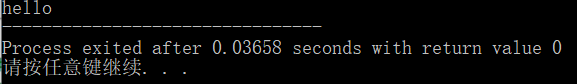## 5.strcat()

函数原型：char *strcat(char *dest, const char *src);

作用：将两个char类型数组相连，结果放在dest中，返回拼接后指向dest的指针。

代码示例

#include<iostream>
#include<string.h>
using namespace std;

int main(){
char s[] = {"hello"};
char s2;

//	将s2数组赋空字符
memset(s2,'\0',10);
//	连接字符数组s和s2
strcat(s2,s);

for(int i=0;i<10;i++){
cout<<s2[i];
}

return 0;
}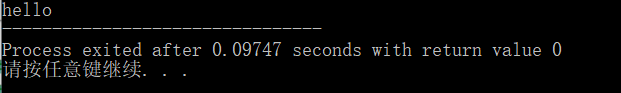## 6.getline()

函数原型：istream& getline ( istream &is , string &str , char delim );

 is 进行读入操作的输入流，常用cin str 已声明的用于存储读入内容的对象，常为string类型 delim 终结符

作用：将输入流is中读到的字符存入str中，直到遇到终结符delim才结束。如果没有定义delim，则默认为 '\n'（换行符）。

注意：getline遇到delim时停止读入（但读入了delim），然后将所读内容存储到string对象中（不存delim）下次读入操作时，将在delim的下个字符开始读入。

代码示例：

#include<stdio.h>
#include<string.h>

int main(){
string str;
getline(cin,str,'o');
printf("%s",str.c_str());  // string类型字符串不能直接使用printf输出，需要调用string中的c_str()函数
}


#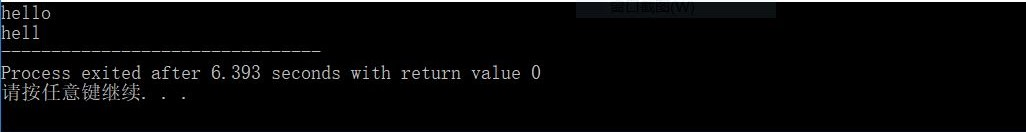## 7.strlen()

函数原型：size_t strlen(const char *string);

作用：计算string字符串或者以char *声明的字符数组的长度。

# <ctype.h>头文件

## 1.tolower()/toupper()

作用：改变字母大小写

例：

char n=’h’;
cout<<(char)toupper(n);      //输出结果为‘H’

注意：n的值并没有改变，仍然为‘h’。

## 2.isalpha（），isdigit（），isprint（）

作用：用来对是否是字母，数字，可打印字符（非控制字符）进行判断

# <math.h>头文件

## 1.pow()

函数原型：double pow(double x, double y)

作用：返回x的y次方

## 2.floor()

函数原型：double floor（double x）；

作用：用于输出浮点类型中小于此数的最大整数

注：floor(n+0.5)可用于进行四舍五入的处理

## 3.ceil()

函数原型：double ceil(double x);

作用：用于输出浮点类型中大于此数的最小整数

## 4.atan()

作用：用来求π的值：atan(1)=π/4    π=4.0*atan（1）

代码示例：

#include<iostream>
#include<math.h>
using namespace std;

// 求π的值，保留小数点后15为小数
int main(){
double pi = 4*atan(1);
printf("%.15f",pi);     //保留π后的十五位小数
}

运行结果：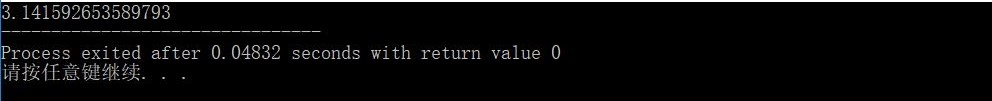# <algorithm>头文件

## 1.min(),max()函数

作用：返回两个元素中最小（最大）的一个

## 2.lower_bound()

函数原型：lower_bound(int* first,int* last,int val);

作用：查找有序区间[first，last]中第一个大于等于val位置

代码示例：

#include<iostream>
#include<algorithm>
using namespace std;

int main(){
int n;
for(int i=0;i<10;i++)
n[i]=i;

cout<<"第一个大于等于7的位置："<<lower_bound(n,n+9,7)<<endl;
cout<<"该位置上的值："<<*lower_bound(n,n+9,7)<<endl;

return 0;
}

运行结果：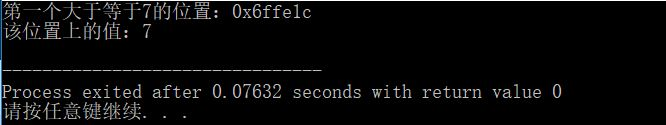## 3.upper_bound（）

作用：大致和lower_bound()相同，不过查找的是有序区间[first，last]中第一个大于x的位置

## 4.next_permutation()/prev_permutation()

格式：next_permutation(数组名，数组名+数组长度)

作用：将数组的排序改为当前数组全排列的下/上一个

返回值：若当前数组存在下/上一个全排列，则返回ture,否则返回false。

解释：假设数组为a,b,c。则该数组的全排列为abc，acb，bac，bca，cab，cba。若当前数组为bac，则其全排列的上一个数组即为acb,下一个数组为bca

注意：若要求数组所有的全排列，需要将数组进行升序排列，否则只能找出该序列之后的全排列数。

代码示例：

#include<iostream>
#include<algorithm>
using namespace std;

//输出abc的全排列
int main(){
char ans={'a','b','c'};
do{
for(int i=0;i<3;++i)
cout<<ans[i]<<" ";
cout<<endl;
}while(next_permutation(ans,ans+3));

return 0;
} 

运行结果：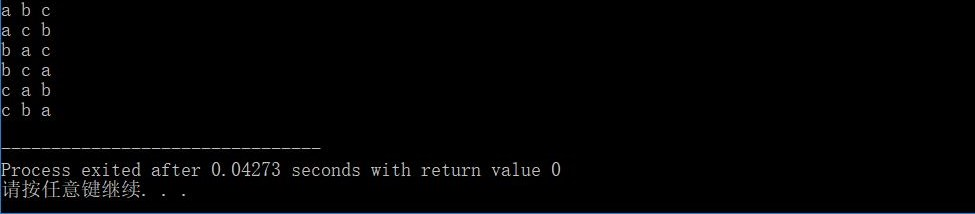## 6.Sort()

函数原型：void sort(RanIt first, RanIt last, Pred pr);

 first 指向容器首地址的指针（数组名） last 指向容器尾地址的指针（数组名+数组长度） pr 比较方法（默认为升序）

具体用法：

sort(begain,end,less<数据类型>())   升序

sort(begain,end,greater<数据类型>()) 降序

注意：开始和结尾用的都是指针

示例代码：

#include<iostream>
#include<algorithm>
using namespace std;

int main(){
char ch={'e','a','c','b','d'};
cout<<"原字符数组为："<<ch<<endl;
//	 升序排列
sort(ch,ch+5);
cout<<"升序排列后为："<<ch<<endl;
//	 降序排列
sort(ch,ch+5,greater<char>());
cout<<"降序排列后为："<<ch<<endl;

return 0;
} 

运行结果：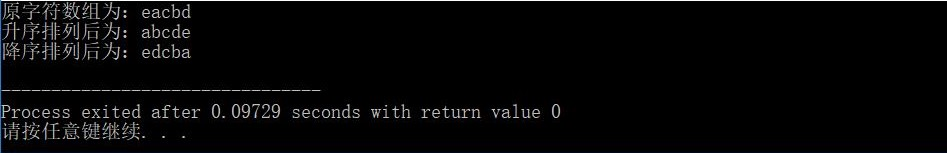# 7.fill()

函数原型：void fill(first,last,val);

 first 起始地址 last 末尾地址 val 将要替换的值

作用：可将数组的值初始化成指定值

例如：

1.初始化一维数组

int a;
fill(a,a+10,80);

2.初始化二维数组

int a;
fill(a,a+10*10,90);

## 8.reverse()

函数原型：void reverse (BidirectionalIterator first, BidirectionalIterator last)

作用：将[first,last)范围内的字符顺序反转

代码示例：

#include <iostream>
#include<algorithm>
#include<cstring>
using namespace std;

int main ()
{
char s1="hello";
string s2="world";

cout<<s1<<endl;
cout<<s2<<endl;

//	reverse的使用
reverse(s1,s1+strlen(s1));
reverse(s2.begin(),s2.end());

cout<<s1<<endl;
cout<<s2<<endl;
}

运行结果：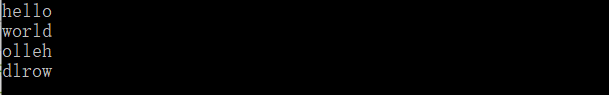展开全文• ## C/C++常用函数汇总

千次阅读 多人点赞 2019-03-20 21:12:29
汇总C/C++中常见的主流函数，适用于竞赛、笔试考前突击
• ## C++常用函数整理

千次阅读 2018-10-18 13:57:00
1. memset 函数解释： 函数原型： 常用形式：
• c/c++常用函数中文参考（c99标准api）。内容包含操作符优先级，ascii码对照表，c语言标准库，c++标准库，c++ STL等。
• C++常用函数及所属库文件，包括函数名称和它的作用。
• c++常用函数， boost， 文件操作， C++常用查询手册
• C++容器vector常用函数 经常使用vector，编程可能会用到vector的各种成员函数，这里总结一下 .size()容量 vector[i] 下标访问 .push_back(X)，为末尾添加元素 .emplace_back(X) 同push_back()，是（内存？）优化...
• 列举了C/C++常用头文件及函数,保存下来可以供随时查阅
• abs()函数和fabs()函数 头文件为cmath，分别用于整数和浮点数的取绝对值 #include <iostream>//输入输出 #include <cmath> using namespace std; const int N = 100010; void main() { int a; ...算法
• tolower()函数是把字符串都转化为小写字母， toupper()函数是把字符串都转化为大写字母 #include <iostream> #include <string> #include <cctype>//tolower、toupper using namespace std; void...
• 主要函数：cin、cout； 使用方法：cin>>变量名； 、cout<<变量名<<endl； //endl代表换行 2.头文件#include<iomanip> 主要函数：setprecision(int n)、setw(int n)、setfill(char c)、...
• max(),min() #include <iostream>//输入输出 #include<algorithm>//max,min using namespace std; void main() { int a; int b; cin >> a >> b; cout <...input.
• 函数原型：double pow(double a,double b); 功能: 计算a的b次方 #include <iostream> #include <math.h>//pow #include <typeinfo>//typeid using namespace std; void main() { int a,b; cin ...算法 c语言
• C++常用库函数（主要是C++标准库中的一些常用库函数的详细讲解和使用方式）
• C/C++ 语言参考 预处理命令 操作符优先级 转义字符 ASCII码表 基本数据类型 关键字 标准 C 库 C++ 标准模板库
• ## C++常用数学函数

千次阅读 2018-07-23 17:42:51
C++中有个头文件math.h ，它是数学函数库,一些数学计算的公式的具体实现是放在math.h里，为了方便大家使用，特在此总结常用的一些函数。 1 三角函数 double sin (double); double cos (double); double tan ...
• //atoi函数 using namespace std; const int N = 100010; void main() { int a; cin >> a; char s; itoa(a, s, 10); cout << s << endl; } 错误 C4996 ‘itoa’: The POSIX name....

# c++常用函数c++ 订阅